# Data handling and visualization using Python

CNRCWP: student workshop

Oleksandr (Sasha) Huziy

UQÀM, December 2013

# Outline part I

• Python basics

• Builtin data types

• Operations on file system, strings and dates

• Modules, classes and functions

• Libraries for scientific computing

• NumPy/SciPy
• Handling NetCDF4 files

• Netcdf4-python

# Syntax

In :
#Variable decalaration <-This is a comment
a = 10; b = "mama"; x = None;

x = [1,2,4, 5, "I am a fifth element"]
#looping
for el in x:
#Checking conditions
if isinstance(el, int):
print(el ** 2 % 10),
else:
msg = ", but my index is {0}."
msg = msg.format(x.index(el))
print(el + msg),

#now we are outside of the loop since no identation
print "\nSomething ..."

1 4 6 5 I am a fifth element, but my index is 4.
Something ...



# Syntax

In :
#accessing elements of a list
x, x[-1], x[1:-1]

Out:
(5, 'I am a fifth element', [2, 4, 5])


## Defining functions

In :
def myfirst_func(x, name = "Sasha"):
"""
This is the comment describing method
arguments and what it actually does
x - dummy argument that demonstrates
use of positional arguments
name - demonstrates use of keyword arguments
"""
print "Hello {0} !!!".format(name)
return 2, None, x


## Calling functions

In :
#Calling the function f
myfirst_func("anything", name = "Oleks")

Hello Oleks !!!


Out:
(2, None, 'anything')


## Defining a class

In :
from datetime import datetime

class Person(object):
#Example of a static class variable
population = 0
def __init__(self, name = "Sasha"):
Person.population += 1
#instance fields
self._birthdate = datetime.now() #private attribute (conv.)
self.name = name #public attribute

def my_moto(self):
return "Go go {0}!!!".format(self.name)

def get_my_age(self):
return datetime.now() - self._birthdate


## Creating and manipulating objects

In :
p1 = Person() #Creating an object of class Person
##Create some more person objects
for i in range(10):
p = Person(name = str(i))

import time
time.sleep(5) #wait for 5 seconds
print p.my_moto()
print "{0} persons were born.".format(p1.population)
print "The age of the first is {0}".format(p1.get_my_age())

Go go 9!!!
11 persons were born.
The age of the first is 0:00:05.000515



# Python basics - code hierarchy

• Each python file is a python module, it can contain function and class definitions

• A folder with python files and a file __init__.py (might be empty file) is called package

# Exercises on basic syntax

• Create a script hello.py in your favourite editor and add print "Hello world", save and run it: python hello.py

• Modify the script making it print squares of odd numbers from 13 to 61 inclusively.

• What will this code print to console:

    x = 1 #define a global variable
def my_function(argument = x):
print argument ** 2
#change the value of the global variable
x = 5
my_function() #call the function defined above


# Builtin data containers

## Python provides the following data structures. Which are actually classes with attributes and methods.

• list (range, *, +, pop, len, accessing list elements, slices, last element, 5 last elements)

• tuple (not mutable, methods)

• dict (accessing elements, keys, size)

• set (set theoretical operations, cannot have 2 equal elements)

# Builtin data containers (lists)¶

In :
#lists (you can conactenate and sort in place)
the_list = [1,2,3,4,5]; other_list = 5 * ;
print the_list + other_list

[1, 2, 3, 4, 5, 6, 6, 6, 6, 6]


In :
#Test if a number is inside a list
print 19 in the_list, 5 in the_list, (6 in the_list and 6 in other_list)

False True False



# Builtin data containers (lists)¶

In :
#square eleaments of a list
#list comprehension
print [the_el ** 2 for the_el in the_list]

[1, 4, 9, 16, 25]


In :
#Generating list or iterable of integers
print range(1,20)

[1, 2, 3, 4, 5, 6, 7, 8, 9, 10, 11, 12, 13, 14, 15, 16, 17, 18, 19]



# Builtin data containers (lists)¶

In :
##There are some utility functions that can be applied to lists
print sum(the_list), \
reduce(lambda x, y: x + y, the_list)

15 15


In :
#loop through several lists at the same time
for el1, el2 in zip(the_list, other_list):
print(el1+el2),

7 8 9 10 11



# Builtin data containers (tuples)

In :
the_tuple = (1,2,3) #tuple is an immutable list, is hashable
print the_tuple[-1]

3



# Builtin data containers (tuples)

In :
#tuples are immutable, e.g:
try:
the_tuple = 25
except TypeError, te:
print te

'tuple' object does not support item assignment



# Builtin data containers (dictionary)

In :
#dictionary
author_to_books = {
"Stephen King":
["Carrie","On writing","Green Mile"],

"Richard Feynman":
["Lectures on computation",
"The pleasure of finding things out"]
}
#add elements to a dictionary
author_to_books["Andrey Kolmogorov"] = \
["Foundations Of The Theory Of Prob..."]


# Builtin data containers (dictionary)

In :
#print the list of authors
print author_to_books.keys()

['Stephen King', 'Richard Feynman', 'Andrey Kolmogorov']


In :
#Iterate over keys and values
for author, book_list in author_to_books.iteritems():
suffix = "s" if len(book_list) > 1 else ""
print("{0} book{1} by {2};\n".format(len(book_list), suffix, author)),

3 books by Stephen King;
2 books by Richard Feynman;
1 book by Andrey Kolmogorov;



# Exercises: builtin containers

• Find a sum of squares of all odd integers that are smaller than 100 in one line of code (Hint: use list comprehensions)

• Find out what does enumerate do.

• Implement recursive fibonacci function with caching, which given the index of a fibonacci number returns its value.

# Modules and classes to operate on

• File system (os, shutil, sys)

• create folder, list folder contents, check if file or folder exists
• Strings (+, *, join, split, regular expressions)

• Dates (datetime, timedelta, )

# File system

In :
import os
#print current directory
print os.getcwd()

/Users/huziy/guziy.github.io/CNRCWP_student_workshop_2013-12-17



# File system

In :
#get list of files in the current directory
flist = os.listdir(".")
print flist[:7]

['.gitignore', '.ipynb_checkpoints', 'countries', 'crsng.png', 'custom.css', 'default_transition.tpl', 'Exercises.html']



# File system

In :
#Check if file exists
fname = flist
print fname,":", os.path.isfile(fname), \
os.path.isdir(fname),  \

.gitignore : True False False



You might also find useful the following modules: sys, shutil, path

# Strings

In :
s = "mama"
#reverse (also works for lists)
print s[-1::-1]

amam


In :
#Dynamically changing parts of a string
tpl = "My name is {0}. I am doing my {1}.\nI am {2} old.\nWeight is {3:.3f} kg"
print tpl.format("Black", "PhD", 25, 80.7823)

My name is Black. I am doing my PhD.
I am 25 old.
Weight is 80.782 kg



# Strings

In :
#Splitting
s = "This,is,a,sentence"
s_list = s.split(",")
print s_list

['This', 'is', 'a', 'sentence']


In :
#joining a list of strings
list_of_fruits = ["apple", "banana", "cherry"]
print "I would like to eat {0}.".format(" or ".join(list_of_fruits))

I would like to eat apple or banana or cherry.



# Strings: regular expressions

In :
#regular expressions module
import re
msg = "Find 192, numbers 278: and -7 and do smth w 89"
groups = re.findall(r"-?\d+", msg)
print groups
print [float(el) for el in groups] #convert strings to floats

['192', '278', '-7', '89']
[192.0, 278.0, -7.0, 89.0]


In :
#regular expressions module
groups = re.findall(r"-?\d+/\d+|-?\d+\.\d+|-?\.?\d+|-?\d+\.?", "Find 192.28940, -2/3 numbers 278: and -7 and .005 w 89,fh.5 -354.")
print groups

['192.28940', '-2/3', '278', '-7', '.005', '89', '.5', '-354']



# Dates

In :
#What time is it
from datetime import datetime, timedelta
d = datetime.now(); print d

2013-12-17 22:25:24.818778


In :
#hours and minutes
d.strftime("%H:%M"), d.strftime("%Hh%Mmin")

Out:
('22:25', '22h25min')


# Dates: How long is the workshop?

In :
start_date = datetime(2013, 12, 17, 9)
end_date = datetime(2013, 12, 18, 17)
print end_date - start_date

1 day, 8:00:00


In :
#you can mutiply the interval by an integer
print (end_date - start_date) * 2

2 days, 16:00:00



# Exercises: Operations with strings, dates and file system

• Get list of all items in the folders from your LD_LIBRARY_PATH environment variable

• Write a function that finds all the numbers in a string using re module (Note: make sure real numbers are also accounted for. Optionally you can also account for the fractions like 2/3)

• Calculate your age in weeks using timedelta

• Figure out on which day of week you were born (see the calendar module), you can have fun by determining on which day of week you'll have your birthday in 2014.

# NumPy

The library for fast manipulations with big arrays that fit into memory.

In :
import numpy as np
#Creating a numpy array from list
np_arr = np.asarray([1,23,4, 3.5,6,7,86, 18.9])
print np_arr

[  1.   23.    4.    3.5   6.    7.   86.   18.9]



# NumPy

In :
#Reshape
np_arr.shape = (2,4)
print np_arr

[[  1.   23.    4.    3.5]
[  6.    7.   86.   18.9]]


In :
#sum along a specified dimension, here
print np_arr.sum(axis = 1)

[  31.5  117.9]



# Numpy

In :
#create prefilled arrays
the_zeros = np.zeros((3,9))
the_ones = np.ones((3,9))

print the_zeros
print 20 * "-"
print the_ones

[[ 0.  0.  0.  0.  0.  0.  0.  0.  0.]
[ 0.  0.  0.  0.  0.  0.  0.  0.  0.]
[ 0.  0.  0.  0.  0.  0.  0.  0.  0.]]
--------------------
[[ 1.  1.  1.  1.  1.  1.  1.  1.  1.]
[ 1.  1.  1.  1.  1.  1.  1.  1.  1.]
[ 1.  1.  1.  1.  1.  1.  1.  1.  1.]]



# Numpy provides many vectorized functions to efficiently operate on arrays

In :
print np.sin(np_arr)

[[ 0.84147098 -0.8462204  -0.7568025  -0.35078323]
[-0.2794155   0.6569866  -0.92345845  0.05042269]]


In :
print np.cross([1,0,0], [0,1,0])

[0 0 1]



# Numpy: fancy indexing

In :
arr = np.random.randn(3,5)
print "Sum of positive numbers: ", arr[arr > 0].sum()

print "Sum over (-0.1 <= arr <= 0.1): ", \
arr[(arr >= -0.1) & (arr <= 0.1)].sum()

print "Sum over (-0.1 > arr) or (arr > 0.1): ", \
arr[(arr < -0.1) | (arr > 0.1)].sum()

Sum of positive numbers:  6.11670510832
Sum over (-0.1 <= arr <= 0.1):  -0.0923470451284
Sum over (-0.1 > arr) or (arr > 0.1):  0.910653974971



# Exercises: Numpy

• Generate a 10 x 3 array of random numbers (in range [0,1]). For each row, pick the number closest to 0.5 (source) Hint: use np.argmin.

• Checkout numpy for MATLAB users transition table.

# SciPy packages

It contains many paackages useful for data analysis

Package Purpose
scipy.cluster Vector quantization / Kmeans
scipy.constants Physical and mathematical constants
scipy.fftpack Fourier transform
scipy.integrate Integration routines
scipy.interpolate Interpolation
scipy.io Data input and output
scipy.linalg Linear algebra routines
scipy.ndimage n-dimensional image package

# SciPy packages (cont.)

It contains many paackages useful for data analysis

Package Purpose
scipy.odr Orthogonal distance regression
scipy.optimize Optimization
scipy.signal Signal processing
scipy.sparse Sparse matrices
scipy.spatial Spatial data structures and algorithms
scipy.special Any special mathematical functions
scipy.stats Statistics

# SciPy: ttest example

In :
from scipy import stats
#generating random variable samples for different distributions
x1 = stats.norm.rvs(loc = 50, scale = 20, size=100)
x2 = stats.norm.rvs(loc=5, scale = 20, size=100)
print stats.ttest_ind(x1, x2)

x1 = stats.norm.rvs(loc = 50, scale = 20, size=100)
x2 = stats.norm.rvs(loc=45, scale = 20, size=100)
print stats.ttest_ind(x1, x2)

(array(15.780486979248632), 7.3369734397094354e-37)
(array(2.0545747739392706), 0.041233488544526832)



# SciPy: integrate a function

$\int\limits_{-\infty}^{+\infty} e^{-x^2}dx \;=\; ?$

In :
from scipy import integrate

In :
def the_func(x):
return np.exp(-x ** 2)
print integrate.quad(the_func, -np.Inf, np.inf)

(1.7724538509055159, 1.4202636780944923e-08)


In :
print "Exact Value sqrt(pi) = {0} ...".format(np.pi ** 0.5)

Exact Value sqrt(pi) = 1.77245385091 ...



# Exercises: SciPy

• Calculate the integral over the cube D=[0,1] x [0, 1] x [0,1] (source):

$\int\int\limits_{D}\int \left(x^y -z \right) dD$

Hint: checkout scipy.integrate.tplquad.

• NetCDF4

• HDF5

# Netcd4-python

## Below is the example of creating a netcdf file using the netcdf4-python library.

In :
from netCDF4 import Dataset
file_name = "test.nc"
if os.path.isfile(file_name):
os.remove(file_name)

#open the file
ds = Dataset(file_name, mode="w")
ds.createDimension("x", 20)
ds.createDimension("y", 20)
ds.createDimension("time", None)
var1 = ds.createVariable("field1", "f4", ("time", "x", "y"))
var2 = ds.createVariable("field2", "f4", ("time", "x", "y"))


# Write actual data to the file

In :
#generate random data and tell to the program where it should go
data = np.random.randn(10, 20, 20)
var1[:] = data
var2[:] = 10 * data + 10
#actually write data to the disk
ds.close();


# Netcdf4-python: reading a netcdf file

In :
from netCDF4 import Dataset

ds = Dataset("test.nc")
#now data is a netcdf4 Variable object, which contain only links to the data
data = ds.variables["field1"]
#now we ask to really read the data into the memory
all_data = data[:]
#print all_data.shape
data1 = data[1,:,:]
data2 = ds.variables["field2"][2,:,:]
print data1.shape, all_data.shape, all_data.mean(axis = 0).mean(axis = 0).mean(axis = 0)

(20, 20) (10, 20, 20) -0.011101



# Exercises: Netcdf4-python

• Demo reading from rpn and saving to netcdf.

The rpn file is /skynet1_rech3/huziy/Converters/NetCDF_converter/monthly_mean_qc_0.1_1979_05.rpn

The variable is PR

# Outline part II

• Plotting libraries

• Matplotlib

• Basemap

• Grouping and subsetting temporal data with pandas

• Interpolation using cKDTree (KDTree) class

• Speeding up your code

# Matplotlib

## The module for creating publication quality plots (mainly 2D), created by John Hunter.

An alternative is PyNGL - a wrapper around NCL developed at NCAR.

# Matplotlib

Example taken from the matplotlib library and modified.

Read some timeseries into memory and import external dependencies:

In :
import matplotlib.pyplot as plt
from datetime import datetime
import matplotlib.cbook as cbook
from matplotlib.dates import strpdate2num
from matplotlib.dates import DateFormatter
from matplotlib.dates import DayLocator, MonthLocator

datafile = cbook.get_sample_data('msft.csv', asfileobj=False)
dates, closes = np.loadtxt(datafile, delimiter=',',
converters={0: strpdate2num('%d-%b-%y')},
skiprows=1, usecols=(0,2), unpack=True)


# Plot the timeseries

In :
fig = plt.figure()
ax.plot(dates, closes, lw = 2);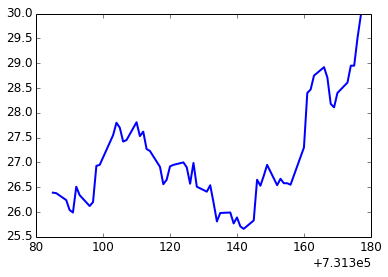# Format the x-axis properly

In :
fig = plt.figure()
ax.plot(dates, closes, lw = 2)

ax.xaxis.set_major_formatter(DateFormatter("%b\n%Y"))
ax.xaxis.set_minor_locator(DayLocator())
ax.xaxis.set_major_locator(MonthLocator())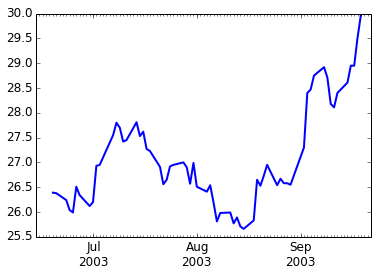# Modify the way the graph looks

In :
def modify_graph(ax):
ax.xaxis.set_major_formatter(DateFormatter("%b"))
ax.xaxis.set_minor_locator(DayLocator())
ax.xaxis.set_major_locator(MonthLocator())
ax.yaxis.set_major_locator(MaxNLocator(nbins=5))
ax.grid()
ax.set_ylim([25.5, 30]); ax.set_xlim([dates[-1], dates]);


# Modify the way the graph looks

In :
fig = plt.figure()
ax.plot(dates, closes, "gray", lw = 3)
modify_graph(ax)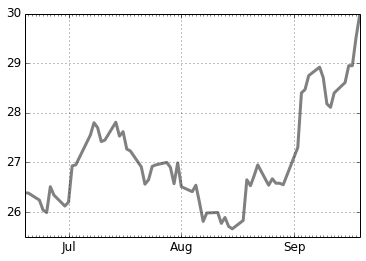# Let us draw the data we just saved to the netcdf file.

Do necessary imports and create configuration objects.

In :
import matplotlib.pyplot as plt
from matplotlib.colors import BoundaryNorm
from matplotlib import cm

levels = [-30,-10, -3,-1,0,1,3,10,30,40]
bn = BoundaryNorm(levels, len(levels) - 1)
cmap = cm.get_cmap("jet", len(levels) - 1);


# Matplotlib: actual plotting

In :
fig, axes = plt.subplots(nrows=1, ncols=3)
im1 = axes.contourf(data1.transpose(),
levels = levels,
norm = bn, cmap = cmap)
im2 = axes.contourf(data2.transpose(),
levels = levels,
norm = bn, cmap = cmap)
apply_some_formatting(axes, im2)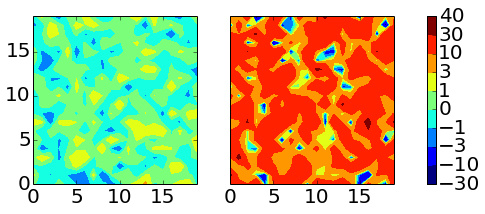# Execises: Matplotlib

• Reproduce the panel plot given in the example. (Try different colormaps)

• Plot a timeseries of daily random data. (Show only month names as Jan, Feb, .. along the x-axis, make sure they do not overlap)

# Load the same timeseries as for matplotlib example

In :
import pandas as pd
datafile = cbook.get_sample_data('msft.csv', asfileobj=False)
df = pd.DataFrame.from_csv(datafile); df.head(3)

Out:
Open High Low Close Volume Adj. Close*
Date
2003-09-19 29.76 29.97 29.52 29.96 92433800 29.79
2003-09-18 28.49 29.51 28.42 29.50 67268096 29.34
2003-09-17 28.76 28.95 28.47 28.50 47221600 28.34

# Select the closes column

In :
closes_col_sorted = df.Close.sort_index()
closes_col_sorted.plot(lw = 2);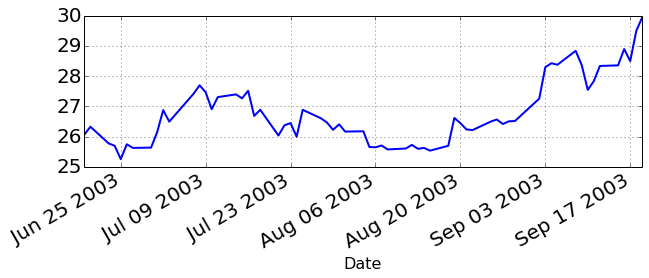# Group by month and plot monthly means

In :
df_month_mean = df.groupby(by = lambda d: d.month).mean()
df_month_mean = df_month_mean.drop("Volume", axis = 1)
df_month_mean.plot(lw = 3);
plt.legend(loc = 2);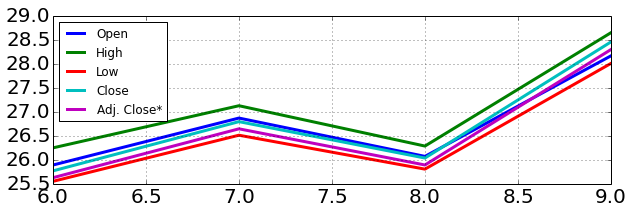# It is easy to resample and do a rolling mean

In :
resampled_closes = closes_col_sorted.resample("5D", how=np.mean)
ax = pd.rolling_mean(resampled_closes, 4)[3:].plot(lw = 2);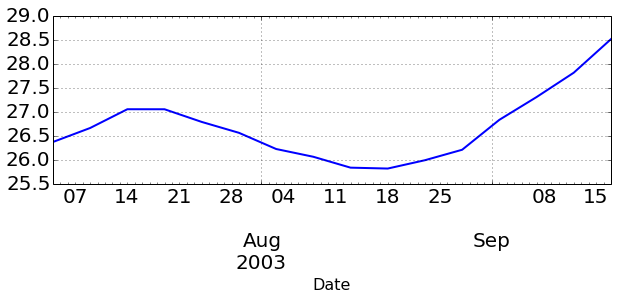# Customizing the graph produced by pandas

In :
ax = pd.rolling_mean(resampled_closes, 4)[3:].plot(lw = 2);
ax.xaxis.set_minor_formatter(NullFormatter())
ax.xaxis.set_major_locator(MonthLocator(bymonthday=1))
ax.xaxis.set_major_formatter(DateFormatter("%b"))
ax.set_xlabel("");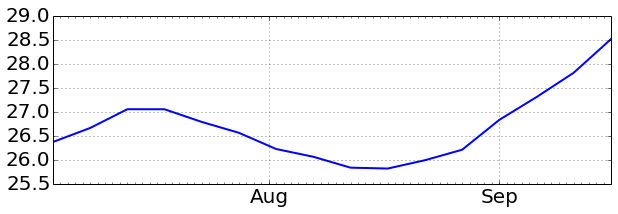# Exercises: Pandas

• Using the same data as in the examples, calculate mean monthly closing prices but using only odd dates (i.e 1st of Jul, 3rd of Jul, ...)

# Basemap

## Example gallery.

PyNGL - can be used as an alternative.

# The usual workflow with the library:

• Create a basemap object
basemap = Basemap(projection="...", ...)

• Draw your field using:
basemap.contour(), basemap.contourf(),
basemap.pcolormesh()

• It provides utility functions to draw coastlines, meridians and shapefiles (only those that contain lat/lon coordinates), mask ocean points.

• Drawing the colorbar is as easy as:
basemap.colorbar(img)


# Basemap

Can be used for nice map backgrounds

In :
from mpl_toolkits.basemap import Basemap
b = Basemap()
fig, (ax1, ax2) = plt.subplots(1,2)

b.warpimage(ax = ax1, scale = 0.1);
im = b.etopo(ax = ax2, scale = 0.1);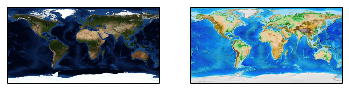In :
import os
if os.path.isfile(local_path):
return

import urllib2
s = urllib2.urlopen(url)
with open(local_path, "wb") as local_file:


# Basemap: display model results in rotated lat/lon projection

In :
f_name = "monthly_mean_qc_0.1_1979_05_PR.rpn.nc"
base_url = "http://scaweb.sca.uqam.ca/~huziy/example_data"
#Fetch the file if it is not there
url = os.path.join(base_url, f_name)

#read the file and check what is inside
ds_pr = Dataset(f_name)
print ds_pr.variables.keys()

[u'rlon', u'rlat', u'level', u'time', u'lon', u'lat', u'rotated_pole', u'preacc']



# Read data from the NetCDF file

In :
rotpole = ds_pr.variables["rotated_pole"]
lon = ds_pr.variables["lon"][:]
lat = ds_pr.variables["lat"][:]
data = ds_pr.variables["preacc"][:].squeeze()
#rotpole.ncattrs(); - returns a list of netcdf attributes of a variable
print rotpole.grid_north_pole_latitude, \
rotpole.grid_north_pole_longitude, \
rotpole.north_pole_grid_longitude

37.8787 106.65 176.709



# Creating a basemap object for the data

In :
lon_0 = rotpole.grid_north_pole_longitude - 180
o_lon_p = rotpole.north_pole_grid_longitude
o_lat_p = rotpole.grid_north_pole_latitude
b = Basemap(projection="rotpole",
lon_0=lon_0,
o_lon_p = o_lon_p,
o_lat_p = o_lat_p,
llcrnrlon = lon[0, 0],
llcrnrlat = lat[0, 0],
urcrnrlon = lon[-1, -1],
urcrnrlat = lat[-1, -1],
resolution="l")
x, y = b(lon, lat) # native coordinates


# Define plotting function to reuse

In :
def plot_data(x, y, data, ax):
im = b.contourf(x, y, data, ax = ax)
b.colorbar(im, ax = ax)
b.drawcoastlines(linewidth=0.5);


# Plot the data

In :
fig, (ax1, ax2) = plt.subplots(1,2)
#plot data as is
plot_data(x, y, data, ax1)
to_plot = np.ma.masked_where(data <= 2, data)
plot_data(x, y, to_plot, ax2)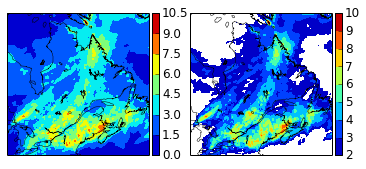# Masking oceans is as easy as

In :
from mpl_toolkits.basemap import maskoceans
lon1 = lon.copy()
lon1[lon1 > 180] = lon1[lon1 > 180] - 360
data_no_ocean = maskoceans(lon1, lat, data)


# Plot masked field

In :
fig, ax = plt.subplots(1,1)
plot_data(x, y, data_no_ocean, ax)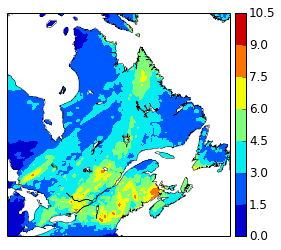In :
#download the shape files directory
local_folder = "countries"
remote_folder = 'http://scaweb.sca.uqam.ca/~huziy/example_data/countries/'
if not os.path.isfile(local_folder+"/cntry00.shp"):
urlpath = urlopen(remote_folder)
pattern = re.compile(r'cntry00\...."')
filelist = pattern.findall(string)
filelist = [s[:-1] for s in filelist if not s.endswith('zip"')]

if not os.path.isdir(local_folder):
os.mkdir(local_folder)
for fname in filelist:
f_path = os.path.join(local_folder, fname)
remote_f_path = os.path.join(remote_folder, fname)


# Basemap: reading and visualizing shape files

In :
bworld = Basemap()
shp_file = os.path.join(local_folder, "cntry00")
ncountries, _, _, _, linecollection = bworld.readshapefile(shp_file, "country")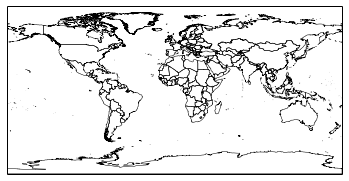# Reading contry polygons (and attributes) from the shape file, necessary imports

In :
import fiona
from matplotlib.collections import PatchCollection
from shapely.geometry import MultiPolygon, shape
from matplotlib.patches import Polygon
import shapely
import brewer2mpl


# Reading country polygons (and attributes) from the shape file, preparations

In :
brew_cmap = brewer2mpl.get_map("Greens", "Sequential", 8)
cmap_shape = brew_cmap.get_mpl_colormap(N = 10)
bounds = np.arange(0, 1.65, 0.15) * 1e9
bn_shape = BoundaryNorm(bounds, len(bounds) - 1)

bworld = Basemap()
populations = []; patches = []

def to_mpl_poly(shp_poly, apatches):
a = np.asarray(shp_poly.exterior)
x, y = bworld(a[:, 0], a[:, 1])
apatches.append(Polygon(zip(x, y)))


# Reading country polygons (and attributes) from the shape file, preparations

In :
with fiona.open('countries/cntry00.shp', 'r') as inp:
for f in inp:
the_population = f["properties"]["POP_CNTRY"]
sh = shape(f['geometry'])

if isinstance(sh, shapely.geometry.Polygon):
to_mpl_poly(sh, patches)
populations.append(the_population)
elif isinstance(sh, shapely.geometry.MultiPolygon):
for sh1 in sh.geoms:
to_mpl_poly(sh1, patches)
populations.append(the_population)


# Coloring the countries based on the attribute value

In :
fig = plt.figure(); ax = fig.add_subplot(111)
pcol = PatchCollection(patches, cmap = cmap_shape, norm = bn_shape)
pcol.set_array(np.array(populations))
cb = bworld.colorbar(pcol, ticks = bounds, format = sf)
cb.ax.yaxis.get_offset_text().set_position((-2,0))
bworld.drawcoastlines(ax = ax, linewidth = 0);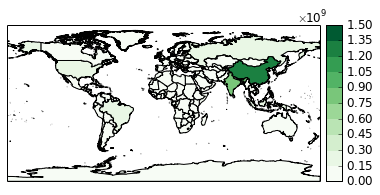# Exercise: shape files

• There is a script on on skynet3 at /skynet3_rech1/huziy/workshop_dir/fiona_test.py which contains a bug. Copy it to your working directory and try to fix the bug.

# Basemap: plotting wind field (read and convert units)

In :
download_link("http://scaweb.sca.uqam.ca/~huziy/example_data/wind.nc", "wind.nc")
ds_wind = Dataset("wind.nc")
u = ds_wind.variables["UU"][:]
v = ds_wind.variables["VV"][:]
coef_wind = 0.51444444444 #m/s in one knot
ds_wind.close()


# Basemap: plotting wind field

In :
from matplotlib.font_manager import FontProperties

In :
fig = plt.figure()
fig.set_size_inches(6,8)
Q = b.quiver(x[::8, ::8], y[::8, ::8],
u[::8, ::8] * coef_wind, v[::8, ::8] * coef_wind)
# make quiver key.
qk = plt.quiverkey(Q, 0.35, 0.1, 5, '5 m/s', labelpos='N',
coordinates = "figure",
fontproperties = dict(weight="bold"))
b.drawcoastlines(linewidth = 0.1);
plt.savefig("wind.jpeg");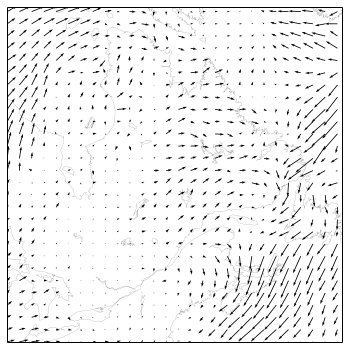# Basemap: plotting wind field (saved image looks better)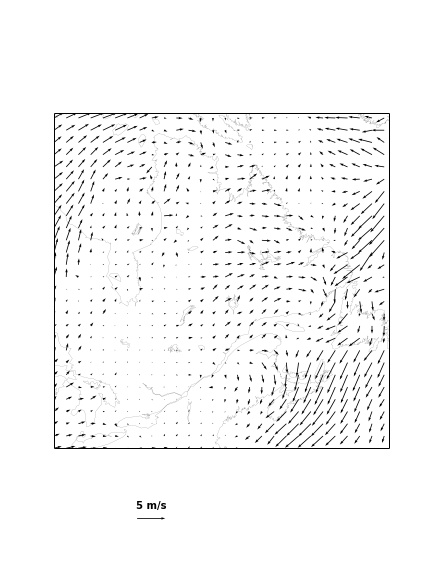# Exercises: Basemap

• Plot fields preacc and air from here using basemap. Add meridians and parallels as well as rivers to the map. Use subplots to put the plots side by side.

• Plot field preacc from here.

# Exercises: Basemap (optional)

• In the model configuration files rotated lat/lon projection is defined using coordinates of the 2 points on a rotated equator so in order to calculate parameters needed for basemap I use this class. The methods of interest are get_north_pole_coords and get_true_pole_coords_in_rotated_system. Try to show model domain using the following information from the model configuration file:
  4   Grd_ni        =   260  ,   Grd_nj          =   260   ,
5   Grd_dx        =    0.1,   Grd_dy          =    0.1 ,
6   Grd_iref      =   142  ,   Grd_jref        =   122   ,
7   Grd_latr      =    0.0,   Grd_lonr        =  180.0 ,
8   Grd_xlat1     =   52,   Grd_xlon1       =  -68,
9   Grd_xlat2     =    0. ,   Grd_xlon2       =   16.65,


# cKDTree

• Is a class defined in scipy.spatial package.

• Alternatives: pyresample and basemap.interp

# cKDTree - my workflow

## 1. Convert all lat/lon coordinates to Cartesian coordinates

    (xs, ys, zs) #correspond to coordinates of the source grid
(xt, yt, zt) #coordinates of the target grid
#All x,y,z - are flattened 1d arrays


## 2. Create a cKDTree object representing source grid

    tree = cKDTree(data = zip(xs, ys, zs))


# cKDTree - my workflow

## 3. Query indices of the nearest target cells and distances to the corresponding source cells


dists, inds = tree.query(zip(xt, yt, zt), k = 1)
#k = 1, means find 1 nearest point


## 4. Get interpolated data (and reshape it to 2D)

    data_target = data_source[inds].reshape(lon_target.shape)


# cKDTree

• For an example usage of cKDTree, please read my post at earthpy.org

• There is a folowup to that post about pyresample here.

# cKDTree: exercise

• Interpolate CRU temperatures to the model grid, use inverse distance weighting for intepolation from 20 nearest points.

$T_t = \frac{\sum_{i=1}^{20}w_i T_{s,i}}{\sum_{i=1}^{20}w_i}$

where $w_i = 1/d_i^2$, $d_i$ - is the distance between corresponding source and target grid points. Grid longitudes and latitudes are defined in this file. The observation temperature field is here.

# Speeding up your code

• Avoid loops and try to make use of Numpy/Scipy/Pandas functionality as much as possible

• If you have a lot of computations the speedup can be achieved by delegating the computations to the NumExpr module.

• You can use Cython to compile libraries to get C-like speed.

• Use multiprocessing module if the tasks are fairly independent and cannot be solved using the above methods

# Multiprocessing example

In :
from multiprocessing import Pool

def func(x):
return x ** 2

p1 = Pool(processes=1)
p2 = Pool(processes=2)

nums = np.arange(100000)


## Make sure it works correctly

In :
print p2.map(func, nums)[:10]

[0, 1, 4, 9, 16, 25, 36, 49, 64, 81]



## Tests of performance gain by using 2 processes instead of 1

In :
%%timeit
p1.map(func, nums)

1 loops, best of 3: 5 s per loop


In :
%%timeit
p2.map(func, nums)

1 loops, best of 3: 3.57 s per loop



# Squaring example using Numpy

In :
%%timeit
sq = np.asarray(nums) ** 2

1000 loops, best of 3: 686 µs per loop



# Squaring example using NumExpr

In :
import numexpr as ne
print ne.detect_number_of_cores()
print ne.evaluate("nums ** 2")[:5]

2
[ 0  1  4  9 16]


In :
%%timeit
ne.evaluate("nums ** 2")

1000 loops, best of 3: 1 ms per loop



# Squaring example using cythonized function

In :
%load_ext cythonmagic

In :
%%cython
def square_list(the_list):
result = []
cdef int i
for i in the_list:
result.append(i ** 2)
return result

In :
%%timeit
square_list(nums)

10 loops, best of 3: 63.3 ms per loop